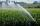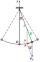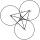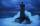# Circle section

Equilateral triangle with side 33 is inscribed circle section whose center is in one of the vertices of the triangle and the arc touches the opposite side.

Calculate:

a) the length of the arc
b) the ratio betewwn the circumference to the circle sector and the perimeter of the triangle

Result

x =  29.928 cm
y =  0.302

#### Solution:Leave us a comment of example and its solution (i.e. if it is still somewhat unclear...):Be the first to comment!#### To solve this example are needed these knowledge from mathematics:

Pythagorean theorem is the base for the right triangle calculator. See also our trigonometric triangle calculator.

## Next similar examples:

1. Equilateral triangleHow long should be the minimum radius of the circular plate to be cut equilateral triangle with side 19 cm from it?
2. Field with vegetablesField planted with vegetables has shape of a rectangular isosceles triangle with leg length of 24 m. At the vertices of the triangle are positioned rotating sprinklers with a range of 12 m. How much of the field sprinkler doesn't irrigated?
3. Chord - TS v2The radius of circle k measures 87 cm. Chord GH = 22 cm. What is TS?
4. PendulumCalculate the length of the pendulum that is 2 cm lower in the lowest position than in the highest position. The length of the circular arc to be described when moving is 20cm.
5. ThalesCalculate the length of the Thales' circle described to right triangle with hypotenuse 18.4 cm.
6. Common chordTwo circles with radius 17 cm and 20 cm are intersect at two points. Its common chord is long 27 cm. What is the distance of the centers of these circles?
7. RT - inscribed circleIn a rectangular triangle has sides lengths> a = 30cm, b = 12.5cm. The right angle is at the vertex C. Calculate the radius of the inscribed circle.
8. CirclesThree circles of radius 95 cm 78 cm and 64 cm is mutually tangent. What is the perimeter of the triangle whose vertices are centers of the circles?
9. HorizonThe top of a lighthouse is 17 m above the sea. How far away is an object which is just “on the horizon”? [Assume the earth is a sphere of radius 6378.1 km.]
10. Perimeter of triangleIn triangle ABC angle A is 60° angle B is 90° side size c is 15 cm. Calculate the triangle circumference.
11. Center traverseIt is true that the middle traverse bisects the triangle?The double ladder shoulders should be 3 meters long. What height will the upper top of the ladder reach if the lower ends are 1.8 meters apart?The double ladder is 8.5m long. It is built so that its lower ends are 3.5 meters apart. How high does the upper end of the ladder reach?The base of the isosceles triangle is 17 cm area 416 cm2. Calculate the perimeter of this triangle.Perimeter of RR triangle (isosceles) is 474 m and the base is 48 m longer than the arms. Calculate the area of this triangle.We want to prove the sentence: If the natural number n is divisible by six, then n is divisible by three. From what assumption we started?Find the reference angle of each angle: